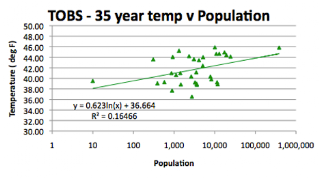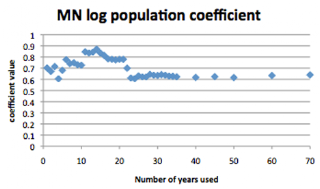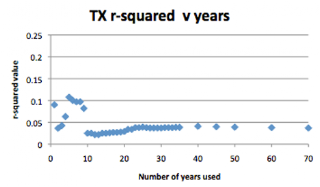## Friday, April 1, 2011

### Temperature correlation with population and the number of years to use

In the last few posts on state historic temperatures, I have changed the basis for the correlation between local station temperature and population from using an average of the station temperature over the whole period to just using the average of the past 30 years. However I chose a 30-year interval without any real basis for the choice. So I thought I would pause the state temperature reviews this week, to see what the best period would be to use for the temperature averages. Because the population data is relatively current (I am using the population given on the citi-data websites) the longer the time interval then the less likely an accurate correlation will be. On the other hand the likely scatter in individual station data might suggest that the longer the time interval the more accurate the correlation.

Note I was about half-way through writing this post when I read the Bishop’s post (Bishop Hill, not mine) on Lord Beddington’s comments on the UHI, specifically
Most stations are not affected by the urban heat island effect and there are well-established ways of taking the effect into account for stations that are (such as comparing temperatures on still and windy nights and excluding urban stations.).
I will have more comment on this statement later in the post, but the results that I am discussing demonstrably show, as I work through them, that the good Lord was wrong. (As I will discuss a little more below). But to return to answering the question first . . .

Starting with the last year of data (2009) I calculated the average temperature for each station and plotted this against the population data. (Though I did this for a number of states I will use the data from Minnesota as the initial state for illustrative purposes).Temperature as a function of station population for Minnesota, using only the last recorded year temperature is reported.

Including a trendline on the plot, I then recorded the constant (35.714), the coefficient (0.7021) and the regression coefficient (0.16893) against the number of years used to calculate the average (in this case one). I then increased the number of years to two, by averaging 2008 and 2009 data, and plotted the curve again. I repeated this, incrementing the number of years in the average by one, until I was averaging 35 years in the plot.Temperature as a function of station population for Minnesota, using an average of the last 35 years for the temperature value.

Beyond this point I stepped the number of years in 5-year increments out to 70 at which point I stopped. Then I plotted the three values in turn against the number of years in the average:The influence of the number of years used in the average on the coefficient of the log of population for Minnesota

One thing to remember is that as more years are included, the added years are likely to be at a lower temperature, but if the temperature change is evenly distributed around the state, then the coefficient should remain the same. If, however, there is a change over time that differs from station to station, then the slope will change over time. Keeping that thought in mind, let’s now look at the change in the constant as the number of years is increased.Variation in the base temperature in the correlation of temperature and population for Minnesota, as a function of the number of years used in determining the average temperature.

The third plot is the correlation coefficient, or r-squared value:The variation in the regression coefficient (r-squared) determined for a plot of temperature with population for Minnesota, as a function of the number of years used to determine the average temperature.

The last plot suggests that (ignoring the one high value at year 22) the best selection for the number of years to include in the calculation is 14. However, before settling on this value I decided to check the values for other states.

The next state, moving backwards in the series, that I checked was Texas. When I had first made these calculations I had included the GISS station data as well as the USHCN station data in the calculation of population effect. One reason for this is that the GISS stations are, by and large, in the cities with the larger populations in each state. But as I have gone round the states in many states at least one of the stations that GISS uses has only data that starts in 1948. This also will affect the overall averages. Texas is not a state that has a good correlation between temperature and population, but, out of curiosity I ran the calculations described above for Texas both combining GISS and USHCN stations, and using only USHCN TOBS data. (I used Texas for this comparative analysis since it has a significant number of GISS stations). The correlation coefficient was significantly better when the GISS data was removed, and so I removed those values from all the correlations.

(This meant, inter alia, redoing the MN calculations, since this was originally calculated including the GISS values – the plots above, and from this point on, will not include the GISS values).

Texas values underwent a significant change where more than 10 years were included, and this is most clearly seen with the r-squared values.The variation in the regression coefficient (r-squared) determined for a plot of temperature with population for Texas, as a function of the number of years used to determine the average temperature.

Part of the reason for this is that there were a couple of stations that only had data until about 2000, and when these were included they reduced the correlation. Without those values the correlation was higher, and interestingly, the coefficient was somewhat similar to that of Minnesota.The influence of the number of years used in the average on the coefficient of the log of population for Texas

The next state that I looked at was Oklahoma, and ran the same procedure to see how changing the number of years included changed the trendline numbers. The plots that I obtained for the regression and population coefficients, using the same procedure, were as follows:The variation in the regression coefficient (r-squared) determined for a plot of temperature with population for Oklahoma, as a function of the number of years used to determine the average temperature.

This would suggest either a dozen or twenty-three years should be used to compute the average temperature, looking at the two peaks. When the population coefficient curve is examined, below, it can be seen that at the point where the highest regression occurs, the value of the coefficient is around 0.65.The influence of the number of years used in the average on the coefficient of the log of population for Oklahoma

The next state, moving steadily North from Oklahoma, is Kansas. This spoils the pattern since, although there are good correlations with latitude and elevation (the other two most significant contributors to varied temperature distribution around the state) there is not a good correlation with population. I did not do as many checks on the number of years for this state, as you can see below, but it is interesting that again there are peaks in the r-squared values at about 10 and 20 year intervals.The variation in the regression coefficient (r-squared) determined for a plot of temperature with population for Kansas, as a function of the number of years used to determine the average temperature.

The correlation coefficient for the log population was about 0.1 in both cases.

Moving North again to Nebraska, one can run the same derivations and get:The variation in the regression coefficient (r-squared) determined for a plot of temperature with population for Nebraska, as a function of the number of years used to determine the average temperature.

Which again suggests that either 10 or 20-22 years to derive an average would be better than 30.The influence of the number of years used in the average on the coefficient of the log of population for Nebraska

Which again gives a coefficient value, in both cases, of between 0.6 and 0.7.

I am not going to belabor the point. In this series I have now looked at the temperature data for just over half of the contiguous states of the Union. It would appear that there is a statistical substantiation for the correlation between temperature and population around the measuring stations of those states of the form:

Temperature = state base temperature + a.logn (local population)

Where a is a constant with a value between 0.5 and 0.8.

I haven’t yet gone back and done the above calculation for all the states yet which would allow a more refined estimate of the value, nor have I yet done the correlation to the three parameters (latitude, elevation and population) together – though it is likely that the latitude and elevation influence the state base temperature, how they interact to control station temperature is not yet clear.

But for now I feel very comfortable stating that Lord Beddington’s statement is demonstrably, factually and terminologically inexact. (To misquote Sir Winston Churchill).

1.شركة كيان لنقل العفش بالرياض والمدينة المنورة وجدة ومكة والطائف والدمام تقديم لكم دليل كامل لشركات نقل العفش بالمملكة العربية السعودية
نقل عفش شركة كيان
دليل شركات نقل العفش
شركة نقل عفش بالمدينة المنورة
شركة نقل اثاث بالرياض
شركة نقل عفش بجدة
شركة نقل عفش بمكة
شركة نقل عفش بالطائف

2.شركة نقل عفش بالرياض وجدة والدمام والخبر والجبيل اولقطيف والاحساء والرياض وجدة ومكة المدينة المنورة والخرج والطائف وخميس مشيط وبجدة افضل شركة نقل عفش بجدة نعرضها مجموعة الفا لنقل العفش بمكة والخرج والقصيم والطائف وتبوك وخميس مشيط ونجران وجيزان وبريدة والمدينة المنورة وينبع افضل شركات نقل الاثاث بالجبيل والطائف وخميس مشيط وبريدة وعنيزو وابها ونجران المدينة وينبع تبوك والقصيم الخرج حفر الباطن والظهران
شركة نقل عفش بجدة
شركة نقل عفش بالمدينة المنورة
شركة نقل اثاث بالرياض
شركة نقل عفش بالدمام
شركة نقل عفش بالطائف
شركة نقل عفش بمكة
شركة نقل عفش بينبع